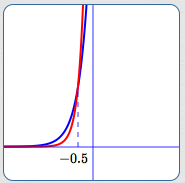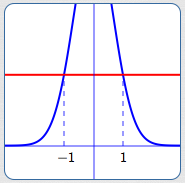﻿ Exponential Functions: Review and Additional Properties

#Exponential Functions: Review and Additional Properties

An exponential function is a function of the form $\,f(x) = b^x\,$ for $\,b \gt 0\,$ and $\,b\ne 1\,.$

Notice that the variable is in the exponent. For example, $\,y = 2^x\,,$ $\,y = (\frac 12)^x\,,$ and $\,y = \text{e}^x\,$ are exponential functions.

Exponential functions and their graphs were introduced in the Algebra II curriculum:

Additional higher-level information that is important for Precalculus is presented below.

## Increasing/Decreasing Properties of Exponential Functions

### Increasing Exponential Functions

When $\,b \gt 1\,,$ $\,f(x) = b^x\,$ is an increasing function. That is, for all real numbers $\,x\,$ and $\,y\,$:

$$\cssId{s13}{x \lt y} \ \ \cssId{s14}{\Rightarrow}\ \ \cssId{s15}{b^x \lt b^y}$$

It is clear from the graph (below) that the other direction is also true:

$$\cssId{s17}{b^x \lt b^y} \ \ \cssId{s18}{\Rightarrow}\ \ \cssId{s19}{x \lt y}$$

Together, we have:

$$\cssId{s21}{x \lt y}\ \ \cssId{s22}{\text{is equivalent to}}\ \ \cssId{s23}{b^x \lt b^y} \tag{1}$$

Notice that when the base is greater than one, the inequality symbols that compare the inputs ($\,x\,$ and $\,y\,$) and their corresponding outputs ($\,b^x\,$ and $\,b^y\,$) have the same direction:

$$\cssId{s26}{x \ {\bf\color{red}{\lt }}\ y}\ \ \cssId{s27}{\text{is equivalent to}}\ \ \cssId{s28}{b^x\ {\bf\color{red}{\lt }}\ b^y} \tag{1a}$$

This equivalence can be alternatively stated as:

$$\cssId{s30}{x \ {\bf\color{red}{>}}\ y}\ \ \cssId{s31}{\text{is equivalent to}}\ \ \cssId{s32}{b^x\ {\bf\color{red}{>}}\ b^y} \tag{1b}$$$y = b^x\,,$ for $\,b \gt 1\,$

An Increasing Exponential Function

$x \lt y \ \ \iff\ \ b^x \lt b^y$

### Decreasing Exponential Functions

When $\,0 \lt b \lt 1\,,$ $\,f(x) = b^x\,$ is a decreasing function. That is, for all real numbers $\,x\,$ and $\,y\,$:

$$\cssId{s39}{x \lt y \ \ \Rightarrow\ \ b^x \gt b^y}$$

It is clear from the graph (below) that the other direction is also true:

$$\cssId{s41}{b^x \gt b^y \ \ \Rightarrow\ \ x \lt y}$$

Together, we have:

$$\cssId{s43}{x \lt y\ \ \text{is equivalent to}\ \ b^x \gt b^y} \tag{2}$$

Notice that when the base is between zero and one, the inequality symbols that compare the inputs ($\,x\,$ and $\,y\,$) and their corresponding outputs ($\,b^x\,$ and $\,b^y\,$) have different directions:

$$\cssId{s46}{x \ {\bf\color{red}{\lt}}\ y\ \ \text{is equivalent to}\ \ b^x\ {\bf\color{red}{\gt}}\ b^y} \tag{2a}$$

This equivalence can be alternatively stated as:

$$\cssId{s48}{x \ {\bf\color{red}{\gt}}\ y\ \ \text{is equivalent to}\ \ b^x\ {\bf\color{red}{\lt}}\ b^y} \tag{2b}$$$y = b^x\,,$ for $\,0 \lt b \lt 1\,$

A Decreasing Exponential Function

$x \lt y \ \ \iff\ \ b^x \gt b^y$

## Exponential Functions are One-to-One

The graphs of exponential functions pass both vertical and horizontal lines tests, so they are one-to-one functions. Thus:

$$\cssId{s56}{x = y \ \ \text{is equivalent to}\ \ b^x = b^y} \tag{3}$$

Consequently, exponential functions have inverses. In a future section, we'll see that the class of logarithmic functions provide inverses to the class of exponential functions.

## Solving Inequalities Involving Exponential Functions

Equivalences (1), (2), and (3) can be used to easily solve certain types of mathematical sentences, as illustrated in the following examples.

### Example 1

Solve:  $2^{3x-1} \lt 2^{5x}$

This is an inequality of the form

$$\cssId{s64}{b^{\text{stuff1}} \lt b^{\text{stuff2}}}$$

where the base, $\,b\,,$ is $\,2\,.$ Notice that the exponential functions on both sides use the same base.

In this example, the base is greater than one, so we'll use equivalence (1):

$$\cssId{s68}{x \lt y \ \ \iff\ \ b^x \lt b^y}$$

Solution:

\begin{align} &\cssId{s70}{2^{3x-1}}\cssId{s71}{\lt} \ \cssId{s72}{2^{5x}}\cr &\quad\cssId{s73}{\text{original inequality}}\cr\cr &\cssId{s74}{3x-1}\cssId{s75}{\lt} \cssId{s76}{5x}\cr &\quad\cssId{s77}{\text{use (1); inequality symbol doesn't change}}\cr\cr &\cssId{s78}{-2x}\cssId{s79}{\lt} \cssId{s80}{1}\cr &\quad\cssId{s81}{\text{addition property of inequality}}\cr\cr &\cssId{s82}{x}\cssId{s83}{\gt} \cssId{s84}{-\frac 12}\cr &\quad\cssId{s85}{\text{divide by a negative #; reverse inequality}} \end{align}Blue curve:  $y = 2^{3x - 1}$
Red curve:  $y = 2^{5x}$
Blue curve lies below red curve for $\,x \gt -\frac 12$

### Example 2

Solve:  $\displaystyle\frac 1{3^{x^2-1}} > 1$

This inequality will be solved in two different ways. As long as you use correct tools in a correct way, there are often different ways you can proceed.

Solution #1:

\begin{align} &\cssId{s96}{\frac 1{3^{x^2-1}}}\cssId{s97}{\gt}\ \cssId{s98}{1}\cr &\quad\cssId{s99}{\text{original inequality}}\cr\cr &\cssId{s100}{\left(\frac 13\right)^{x^2-1}}\cssId{s101}{\gt}\cssId{s102}{\left(\frac 13\right)^0}\cr &\quad\cssId{s103}{\text{rename both sides using exponent laws}}\cr\cr &\cssId{s104}{x^2 - 1}\cssId{s105}{\lt} \cssId{s106}{0}\cr &\quad\cssId{s107}{\text{use (2); inequality changes direction}}\cr\cr &\cssId{s108}{x^2}\cssId{s109}{\lt} \cssId{s110}{1}\cr &\quad\cssId{s111}{\text{addition property of inequality}}\cr\cr &\cssId{s112}{-1} \cssId{s113}{\lt}\cssId{s114}{x} \cssId{s115}{\lt} \cssId{s116}{1}\cr &\quad\cssId{s117}{\text{inspection (knowledge of x^2 curve)}} \end{align}

Solution #2:

\begin{align} &\cssId{s119}{\frac 1{3^{x^2-1}}}\cssId{s120}{\gt} \ \cssId{s121}{1}\cr &\quad\cssId{s122}{\text{original inequality}}\cr\cr &\cssId{s123}{1}\cssId{s124}{\gt}\cssId{s125}{3^{x^2-1}}\cr &\quad\cssId{s126}{\text{multiply both sides by 3^{x^2-1}\overset{\text{always}}{\gt}0}}\cr\cr &\cssId{s127}{3^0} \cssId{s128}{\gt}\cssId{s129}{3^{x^2-1}}\cr &\quad\cssId{s130}{\text{rename: 1 = 3^0}}\cr\cr &\cssId{s131}{0}\cssId{s132}{\gt}\ \cssId{s133}{x^2 - 1}\cr &\quad\cssId{s134}{\text{use (1)}}\cr\cr &\cssId{s135}{x^2}\cssId{s136}{\lt}\cssId{s137}{1}\cr &\quad\cssId{s138}{\text{addition property; re-arrange}}\cr\cr &\cssId{s139}{-1} \cssId{s140}{\lt}\cssId{s141}{x}\cssId{s142}{\lt} \cssId{s143}{1}\cr &\quad\cssId{s144}{\text{inspection (knowledge of x^2 curve)}} \end{align}Blue curve:  $\displaystyle y = \frac{1}{3^{x^2-1}}$
Red curve:  $y = 1$
Blue curve lies above red curve for $\,x\,$ between $\,-1\,$ and $\,1\,$